Equations > Geometry > Area

Showing results 1 to 10 of 15, on page 1 of 2
Go to page: 1 2
 Description Equation Area of a circle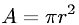Area of an ellipse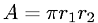Area of an equilateral triangleArea of a parallelogram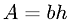Area of a rectangle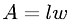Area of a regular polygon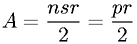Area of a rhombus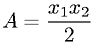Area of a sector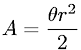Area of a square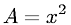Area of a trapezoid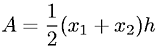(you need to login first) Go to page: 1 2